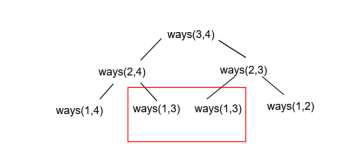# Count number of ways to partition a set into k subsets in C++

C++Server Side ProgrammingProgramming

Given two numbers e and p. The goal is to count the number of ways in which we can divide e elements of a set into p partitions/subsets.

For Example

## Input

e=4 p=2

## Output

Count of number of ways to partition a set into k subsets are: 7

## Explanation

If elements are: a b c d then ways to divide them into 2 partitions are: (a,b,c)−(d), (a,b)−(c,d), (a,b,c)−(d), (a)−(b,c,d), (a,c)−(b,d), (a,c,d)−(b), (a,b,d)−(c). Total 7 ways.

## Input

e=2 p=2

## Output

Count of number of ways to partition a set into k subsets are: 1

## Explanation

If elements are: a b then ways to divide them into 2 partitions are:
(a)− (b). Total 1 way only.

Approach used in the below program is as follows

In this approach we will use a dynamic programming approach. The calculations used in the solution would always be recursive. If we divide elements into p partistions then −

• If e−1 elements can be divided into p partitions in ways(e-1,p). Then we can put current element into one of these p partitions in p*ways(e-1,p).

• If e−1elements are divided into p−1 partitions in ways(e−1,p−1) then putting 1 element in that separate 1 partition will have 1*ways(e−1,p−1). Total ways will be p*ways(e−1,p)+ways(e−1,p−1). This method would become recursive −As shown above duplicate computations will be done. To avoid this we will use a dynamic programming approach.

• Take variables, elements and partitions as input.

• Function partition_k(int elements, int partition) takes both variables and returns the count of the number of ways to partition a set into k subsets.

• Take 2D array arr[elements + 1][partition + 1] to store values of ways(e,p) in arr[e][p].

• Using a for loop from i=0 to i=elements, set arr[i] = 0 as the number of partitions is 0 then ways(i,0)=0.

• Again using a for loop from j=0 to i=partitions, set arr[j] = 0 as number of elements is 0 then ways(0,i)=0.

• Now traverse arr[][] using two for loops from i=1 to i<=elements and j=1 to j<=i. and fill rest values.

• For a single element, ways=1 or for dividing x elements into x partitions there is only 1 way. So set arr[i][j] = 1 in case i==j or j==1.

• Otherwise set temp_1 = arr[i−1][j−1] and temp_2 = arr[i−1][j] and update arr[i][j] = j * temp_2 + temp_1.

• At the end of all loops we will have arr[elements][partition] as total ways.

• Return arr[elements][partition] as result.

## Example

Live Demo

#include<iostream>
using namespace std;
int partition_k(int elements, int partition){
int arr[elements + 1][partition + 1];
for(int i = 0; i <= elements; i++){
arr[i] = 0;
}
for(int j = 0; j <= partition; j++){
arr[partition] = 0;
}
for(int i = 1; i <= elements; i++){
for (int j = 1; j <= i; j++){
if (j == 1 || i == j)
{ arr[i][j] = 1; }
else{
int temp_1 = arr[i−1][j−1];
int temp_2 = arr[i−1][j];
arr[i][j] = j * temp_2 + temp_1;
}
}
}
return arr[elements][partition];
}
int main(){
int elements = 4;
int partition = 2;
cout<<"Count of number of ways to partition a set into k subsets are: "<<partition_k(elements, partition);
return 0;
}

## Output

If we run the above code it will generate the following output −

Count of number of ways to partition a set into k subsets are: 7